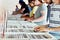# Applying binary search in JavaScriptPhoto by Anthony Martino on Unsplash

# Starting from linear search

`const arr = [2,4,3,5,7,6,8,5,10]let target = 8for(let i = 0; i < arr.length; i++){// do something with arr[i] to find value 8 in the array}`

# Binary Search explained

`// STEP 1first sort the array and establish a given target to search for// STEP 2define the current search area and its midpoint// STEP 3repeat the following until we either find 8 or run out of search area:  a. check if the value in this point equals the target  b. if it does, great we found it!        c1. if the midpoint is greater than the target, halve the search area by searching to the left of the midpoint  c2. if the midpoint is lower than the target, halve the search area by searching to the right of the midpoint`

# Simple Binary Search in JavaScript

## Step 1: Sort the array and establish a given target to search for

`const arr = [12,5,7,3...];const sortedArray = arr.sort()// [1,3,5,5,7,8,11,12,14,14,15,16,16,17,17,17,17,18,19,19,19];let target = 18;`

# Step 2: Define the current search area

`const sortedArray = [1,3,5,5,7,8,11,12,14,14,15,16,16,17,17,17,17,18,19,19,19];let target = 18;// start index of the array we are searching inlet start = 0;// end index of the array we are searching in let end = arr.length - 1;// midpoint to check for the target let middle = Math.floor(start + end / 2);`

# Step 3: Repeat the “checking-halving” logic until we either succeed or run out of search area

`const sortedArray = [1,3,5,5,7,8,11,12,14,14,15,16,16,17,17,17,17,18,19,19,19];let target = 18;let start = 0;let end = arr.length - 1;let middle = Math.floor(start + end / 2);// a. check if the value in this point equals the targetif(arr[middle] === target){  // b. if it does, great we found it!  return true;      }  // c1. if the midpoint is greater than the target, halve the search area by searching to the left of the midpointelse if(arr[middle] > target){          end = middle - 1      }// c2. if the midpoint is lower than the target, halve the search area by searching to the right of the midpointelse if(arr[middle] < target) {         start = middle + 1;}`

# The binary Search runtime

I’m a product manager and developer. I’ve just started writing about what I learn and find fascinating.

## More from Stephanos Theodotou

I’m a product manager and developer. I’ve just started writing about what I learn and find fascinating.